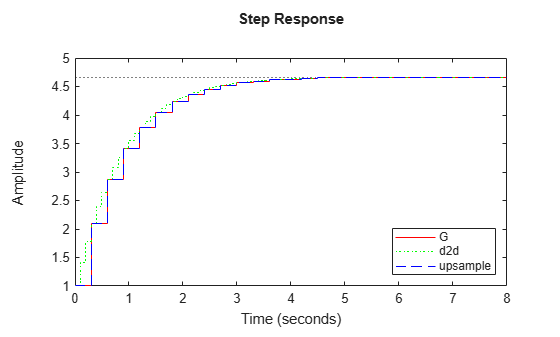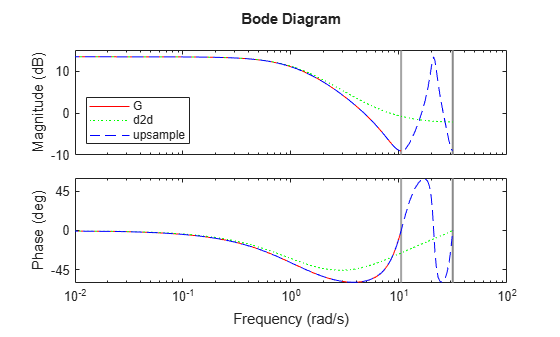# Upsample Discrete-Time System

This example shows how to upsample a system using both the `d2d` and `upsample` commands and compare the results of both to the original system.

Upsampling a system can be useful, for example, when you need to implement a digital controller at a faster rate than you originally designed it for.

Create the discrete-time system

`$G\left(z\right)=\frac{z+0.4}{z-0.7}$`

with a sample time of 0.3 s.

`G = tf([1,0.4],[1,-0.7],0.3);`

Resample the system at 0.1 s using `d2d`.

`G_d2d = d2d(G,0.1)`
```G_d2d = z - 0.4769 ---------- z - 0.8879 Sample time: 0.1 seconds Discrete-time transfer function. ```

By default, `d2d` uses the zero-order-hold (ZOH) method to resample the system. The resampled system has the same order as `G`.

Resample the system again at 0.1 s, using `upsample`.

`G_up = upsample(G,3)`
```G_up = z^3 + 0.4 --------- z^3 - 0.7 Sample time: 0.1 seconds Discrete-time transfer function. ```

The second input, `3`, tells `upsample` to resample `G` at a sample time three times faster than the sample time of `G`. This input to `upsample` must be an integer.

`G_up` has three times as many poles and zeroes as `G`.

Compare the step responses of the original model `G` with the resampled models `G_d2d` and `G_up`.

```step(G,'-r',G_d2d,':g',G_up,'--b') legend('G','d2d','upsample','Location','SouthEast')```The step response of the upsampled model `G_up` matches exactly the step response of the original model `G`. The response of the resampled model `G_d2d` matches only at every third sample.

Compare the frequency response of the original model with the resampled models.

```bode(G,'-r',G_d2d,':g',G_up,'--b') legend('G','d2d','upsample','Location','SouthWest')```In the frequency domain as well, the model `G_up` created with the `upsample` command matches the original model exactly up to the Nyquist frequency of the original model.

Using `upsample` provides a better match than `d2d` in both the time and frequency domains. However, `upsample` increases the model order, which can be undesirable. Additionally, `upsample` is only available where the original sample time is an integer multiple of the new sample time.